# 黑盒模型事后归因解析：LIME方法

2020 年 4 月 26 日## 2. LIME 介绍

LIME 全称 Local Interpretable Model-Agnostic Explanations，由 Marco Ribeiro, Sameer Singh 和 Carlos Guestrin 三个人在 2016 年《“Why Should I Trust You?” Explaining the Predictions of Any Classififier》这一文中提出来的，是一种事后解释方法，即在建立模型之后所做的解释，其名称也很好的反应了它的特点：

Local: 基于想要解释的预测值及其附近的样本，构建局部的线性模型或其他代理模型；

Interpretable: LIME 做出的解释易被人类理解。利用局部可解释的模型对黑盒模型的预测结果进行解释，构造局部样本特征和预测结果之间的关系；

Model-Agnostic: LIME 解释的算法与模型无关，无论是用 Random Forest、SVM 还是 XGBoost 等各种复杂的模型，得到的预测结果都能使用 LIME 方法来解释；

Explanations: LIME 是一种事后解释方法。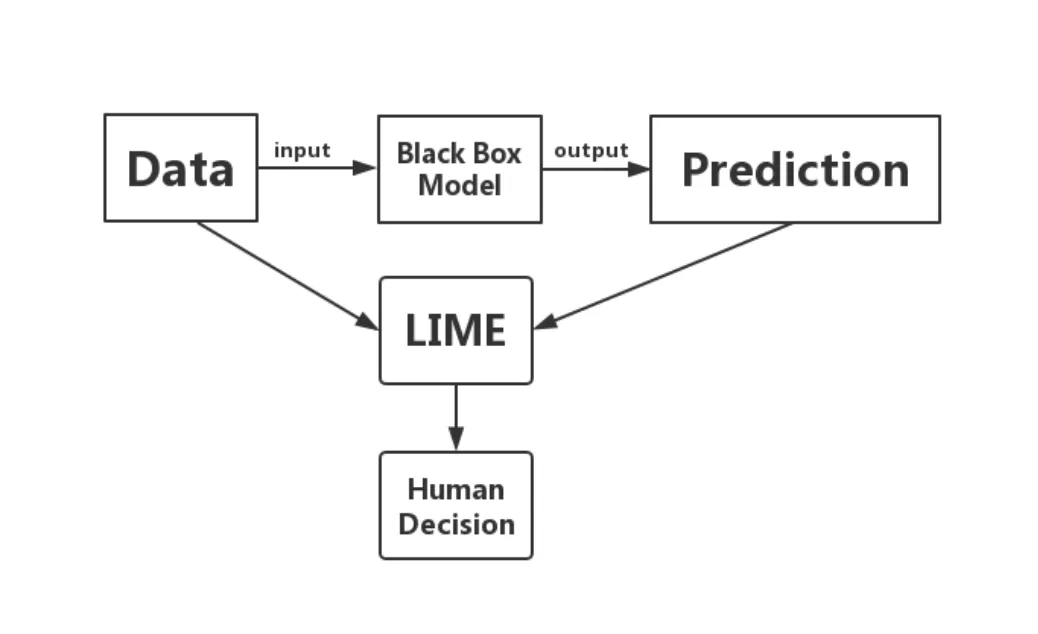LIME 可处理不同类型的输入数据，如表格数据 (Tabular Data)、图像数据（Image Data）或文本数据 (Text Data)。对于表格数据，如用银行客户行为数据预测理财产品销售，训练完复杂模型后可以用 LIME 得到哪些特征影响理财产品销售；图像数据，如识别图片中的动物是否为猫，训练完复杂模型后可以用 LIME 得到图片中的动物被识别为猫是因为哪一个或几个像素块；文本数据，如识别短信是否为垃圾短信，训练完复杂模型后可以用 LIME 得到一条信息被判断为垃圾短信是因为哪一个或几个关键词。

## 3. LIME 原理

LIME 的算法需要输入想要解释的预测样本和已经训练好的复杂模型，基于表格数据，算法步骤如下：

（1）预测样本附近随机采样：对于连续型 (continuous) 特征，LIME 在预测样本点附近用一个标准正态分布 N(0,1) 来产生指定个数（代码中设置的 num_samples）的样本；而对于类别型 (categorical) 特征，则根据训练集的分布进行采样，当新生成样本的类别型特征与预测样本相同时，该类别型特征取值为 1，否则取值为 0；假设，想要解释的预测点为 x=(x1,x2,,xp)$x^{*}=(x_1,x_2,\dots,x_p)$ , 有 p 个特征, 其中第 i 个特征为连续型特征，取值为 $x_i$$\sigma_{i}$ 为 该特征在训练集中的标准差；生成的 N 个样本为 $z_k=(z_1,z_2,\dots,z_p), k=1,2,\dots ,N$, 通过标准正态分布 $N(0,1)$ 对应生成一个随机数 $a_{ki}$（对应第 k 个新生成样本的第 i 个特征），则新生成的第 k 个样本的第 i 个特征的取值为 $a_{ki}*\sigma_{i}+x_{i}$

（2）对新生成的样本打标签：将新生成的样本放入已经训练好的复杂模型中训练，得到对应的预测结果；设训练好的复杂模型为 $f$，则新生成的的样本预测结果为 $f(z_1),f(z_2),\dots,f(z_N)$

（3）计算新生成的样本与想要解释的预测点的距离并得到权重：新生成的样本距离想要解释的预测点越近，我们认为这些样本能够更好的解释预测点，因此需要赋予更高的权重。我们用指数核函数（exponential kernal）去定义新生成样本的权重，设为 $\Pi_{x^{*}}(z)=exp (\frac{-D(x^{*},z)^{2}}{\sigma^{2}})$, 此处 $D(x^{*},z)$ 为某个新生成的样本到 $x^{*}$ 的距离函数， $\sigma$ 则为超参数。从公式中可以看到，距离越近， $\Pi_{x^{*}}$ 的值越大。

（4）筛选用来解释的特征，拟合线性模型：设想要用来解释的特征有 $p^{’}$ 个，则用来解释的特征为 $z^{’}=(z_{(1)},z_{(2)},\dots,z_{(p^{’})})$，此处 $z_{(1)}$$z_{1}$ 不一定相等，只是用来表示从 p 个特征中选取 $p^{’}$ 个作为解释；设用来解释的线性模型为 $g(z^{’})=\omega_{g}.z^{’}=\omega_{0}+\omega_{1}z_{(1)}+\dots \omega_{p^{’}}z_{(p^{’})}$ ，为了求出线性模型的系数，我们用一个加权平方损失构造损失函数：$\mathcal{L}(f,h,\Pi_{x^{*}})=\sum_{k=1}^{N} \Pi_{x^{*}}(z_{k})(f(z_{k})-g(z^{’}_{k}))^2$, 找出使得损失最小的 $\omega_{g}$ ，而 $\omega_{g}.z^{’}$ 即为我们用来解释的特征的线性组合；而对于 $p^{’}$ 个特征的选择，代码中提供 forward selection、highest weights、lasso_path 等方法。

## 4. Submodular Pick

### 4.1 什么是 submodular?

submodular（次模）实际上是对“边际效用递减”这个说法的形式化。对于一个集合函数 $f: 2^{V} \rightarrow R$, 如果有一个集合 V，满足 $V \subseteq S$, 那么在 $V$ 中增加一个元素所增加的收益要小于等于在 $V$ 的子集中增加一个元素所增加的收益。更清晰地表示：对于函数 $f$ 而言，若 $A \subseteq B \subseteq S$, 且 $e \in S-B$，则 $f(B \cup \lbrace e \rbrace)-f(B) \leq f(A \cup \lbrace e\rbrace)-f(A)$

### 4.2 Submodular pick(SP) 的步骤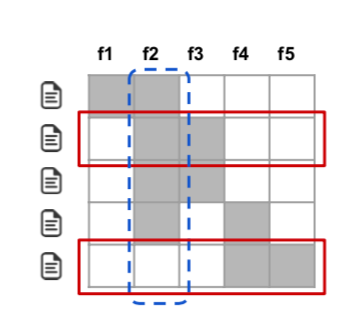$c(V,W,I)=\sum_{j=1}^{d^{’}} 1_{\exists i \in V:W_{ij} >0} I_j$

$Pick(W,I)=argmax_{V,|V|\leq n} c(V,W,I)$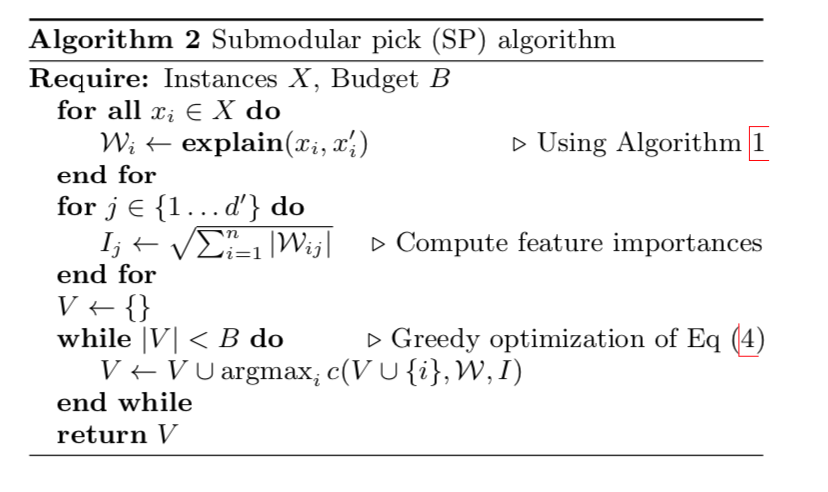## 5. 案例展示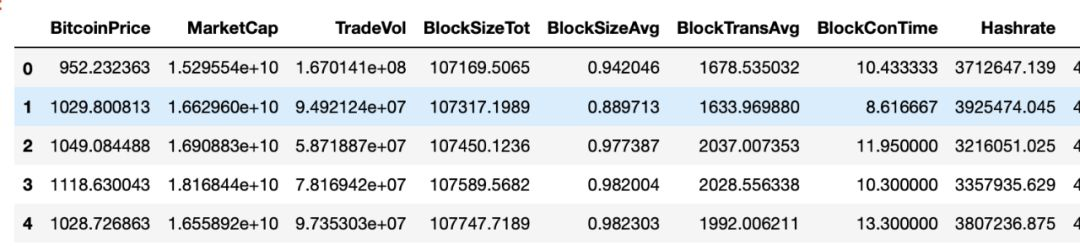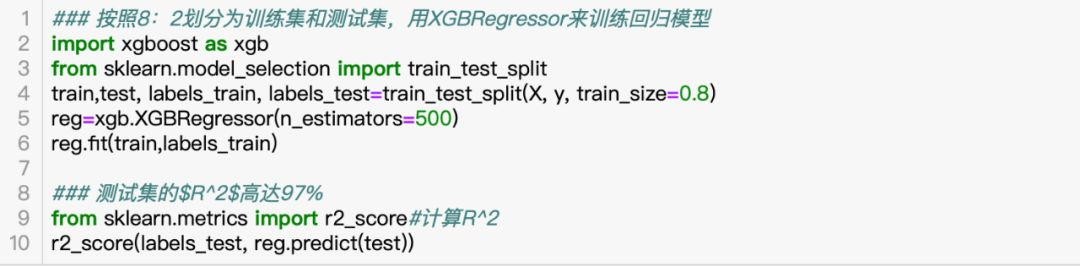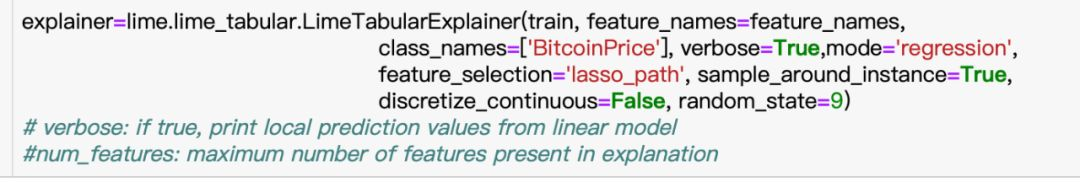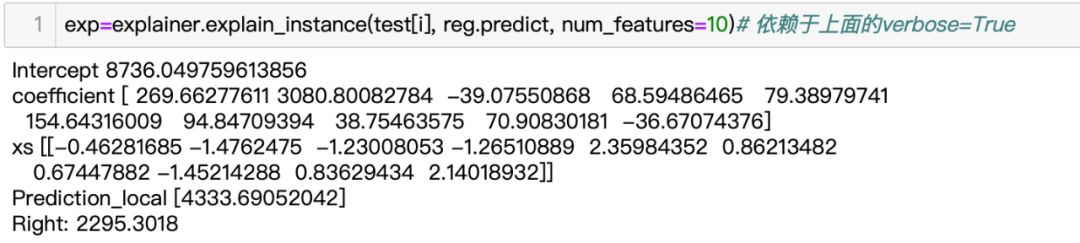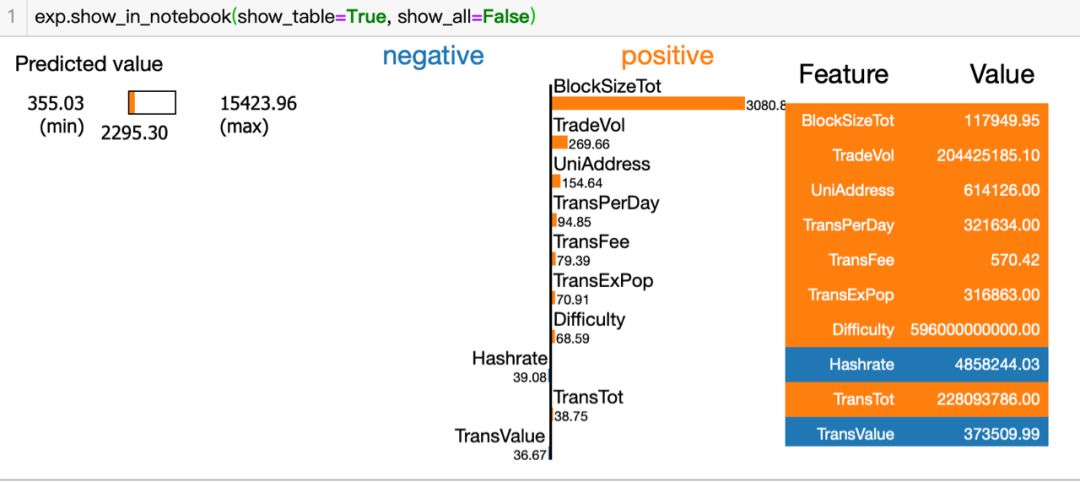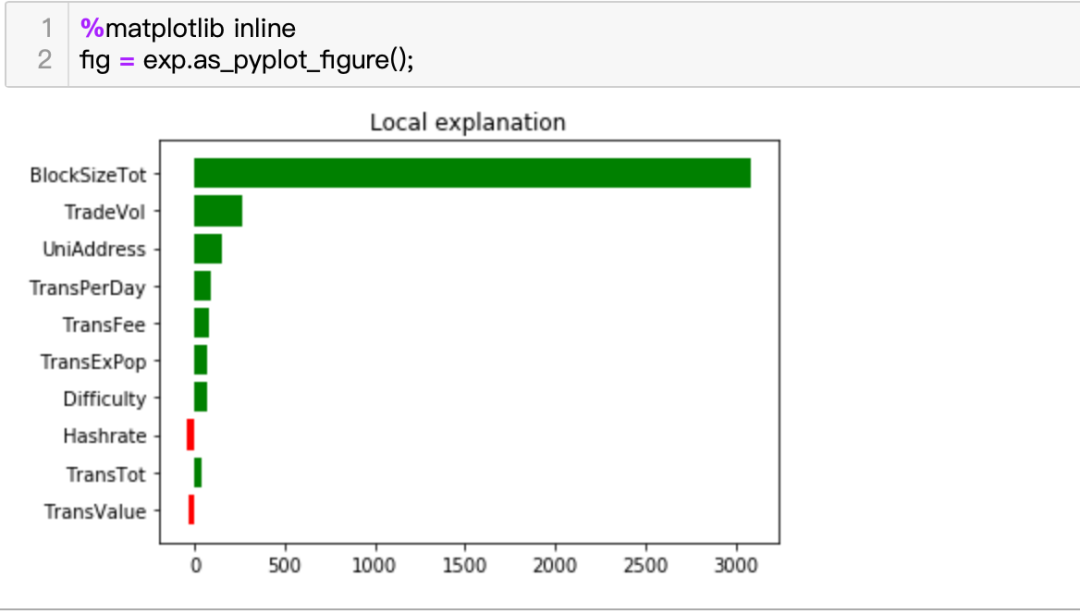from lime import submodular_picksp_obj = submodular_pick.SubmodularPick(explainer, train, reg.predict, sample_size=10, num_features=10, num_exps_desired=1)[exp.as_pyplot_figure() for exp in sp_obj.sp_explanations]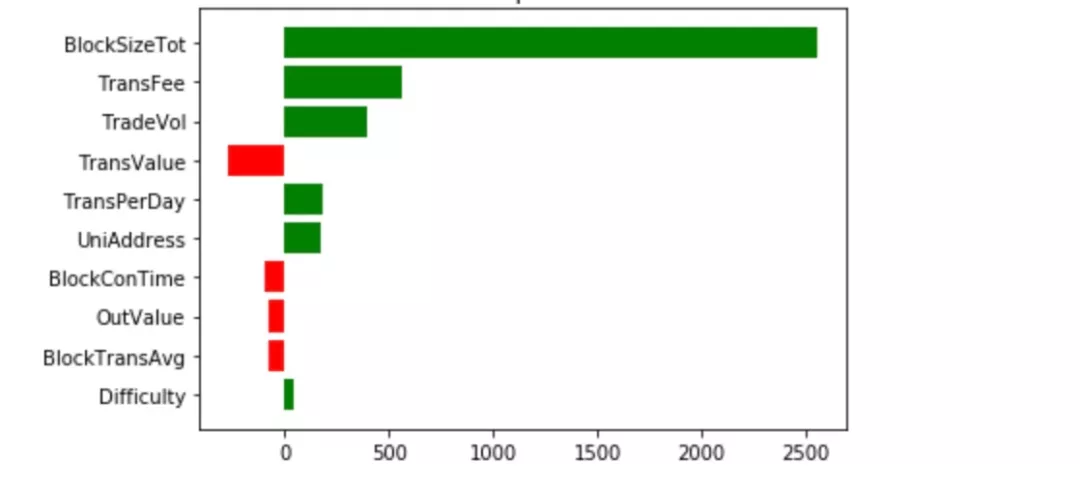## 7. 参考文档

 Túlio Ribeiro, M., Singh, S., Guestrin, C.: “Why Should I Trust You?”: explaining the predictions of any classifier. In: ACM SIGKDD International Conference on Knowledge Discovery and Data Mining (2016)

2020 年 4 月 26 日 18:27 1540

## 评论

• ###### 机器学习笔记（二）：线性回归

本文为华为云系列文章之一。

• ###### 黑盒模型事后归因解析：SHAP 方法

我们希望可以通过一系列文章来介绍解释不同模型的方法，并分享与实际场景相结合的业务与技术心得。

• ###### 模型的分类方式

机器学习模型都有哪些分类呢？

2018 年 6 月 14 日

• ###### 7 种机器学习算法的 7 个要点

我们可能会忽略一些算法背后的关键概念或思想，而这些概念或思想对于这些算法的理解是必不可少的。

• ###### 机器学习笔记（一）：基本概念

本文是作者在机器学习方面的学习笔记。

• ###### 28 丨 EM 聚类（上）：如何将一份菜等分给两个人？

我们先看一个简单的场景：假设你炒了一份菜，想要把它平均分到两个碟子里，该怎么分？

2019 年 2 月 15 日

• ###### 08 机器学习 | 简约而不简单：线性回归

数学中的线性模型可谓“简约而不简单”：它既能体现出重要的基本思想，又能构造出功能更加强大的非线性模型。

2017 年 12 月 26 日

• ###### 自适应的基函数：神经网络

今天我们来讨论神经网络的基本原理，我们从感知器聊起。

2018 年 7 月 24 日

#### 《架构师训练营》第六周总结